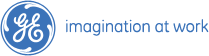# Placement Papers - GEGE PAPER - 30 JUN 2007 - CALICUT
Posted by :
Jana
(28)
Hi Friends

First section consisted of 10 aptitude questions
1. pipes and cistern problem
2. ratio and proportion problem
3. to find the angle (triangles, circles) (2questions)
4. non verbal reasoning
5. 2^x = 7^y = 14^z, then express z in terms of x and y
6. men and work
7. boat and stream
8. a simple coding problem

technical-mechanical-stress analysis-10 questions
1. shear force diagram simply supported beam with an eccentric moment
2. failure theory for ductile materials
3. stress due to temperature change in free bar
4. where is the stress accurate in a finite element? (options: node, interior, gauss point, centroid)
5. hoop stress problem
6. mohr circle representation

Remya